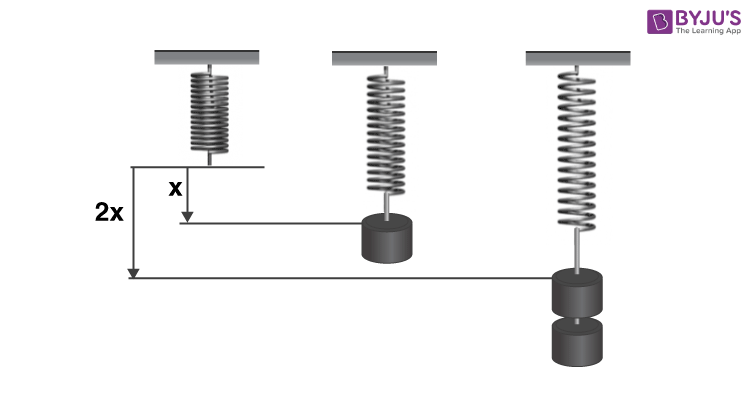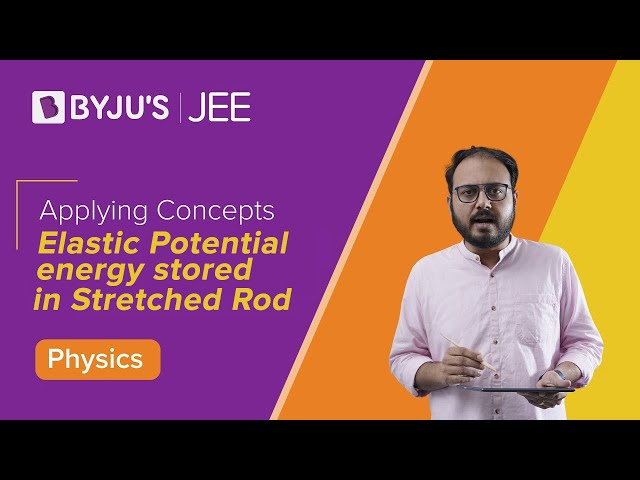# Elastic Potential Energy And Spring Potential Energy

Elastic potential energy and spring potential energy are one of the most interesting concepts in physics. In this article, we will learn about the concepts of these topics in a detailed manner.

## What is Potential Energy?

Potential energy is the energy possessed by a body by virtue of its position with reference to a position zero. The two common types of  potential energy are:

• Gravitational Potential Energy
• Elastic Potential Energy

## Elastic Potential Energy

Elastic Potential Energy is the potential energy stored in an elastic material when it is stretched or compressed. The amount of energy stored is proportional to the amount stretched or compressed, as in, the more the amount of stretch, the greater is the energy stored.

Have you tried jumping on a trampoline? Or ever seen someone, do it? Ever noticed that the more the trampoline stretches downwards when a person jumps on it, the more they are thrown upwards? This is an example of elastic potential energy.

Check out the picture given below.Among the three springs, which one do think has more potential energy? Quite clearly, the third one as it has been stretched by the greatest amount (2x), compared to the other two (0 and x). By virtue of its position, the third spring has the greatest amount of elastic potential energy in this case. You can also think of it this way. The amount of work done in stretching the spring is just being converted into energy here.

Based on Hooke’s Law, we already know that for elastic materials, the force applied by the spring is proportional to its displacement, given by the following relation.

$$F$$ =$$kx$$

where,

x is the displacement (stretch or compression)
k is the spring constant

At the equilibrium position of such a system (where the force applied is 0), the potential energy possessed by the system is zero. To calculate the potential energy stored in the spring when it is displaced by a certain amount, the following formula can be used.

$$U$$ = $$\frac{1}{2}kx^2$$

where,
U is the elastic potential energy of the system

### Spring Potential Energy

When a stretched spring is compressed or extended, we experience a force that is equal to the force applied by us in the opposite direction. But as soon as the stress is relieved, instantly the spring gains its normal shape. This is called as the spring potential energy. The elastic potential energy of the spring helps to get back to normal shape. Generally, it follows Hooke’s law.

The video explains how a stretched rod is analogous to a stretched spring.### What is Hooke’s law?

According to this Hooke’s  Law, the force which is needed to change the shape of the spring is proportional to the spring’s displacement. Here, displacement is how far the spring is stretched or compressed from its original shape.

We can mathematically summarize Hook’s Law as:

F= – k x

Where,

‘k’ is the spring constant

‘x’ is the displacement.

#### Related Physics Articles

Stay tuned to BYJU’S to learn interesting physics topics with the help of engaging video lessons.

Test Your Knowledge On Elastic Potential Energy Spring Potential Energy!